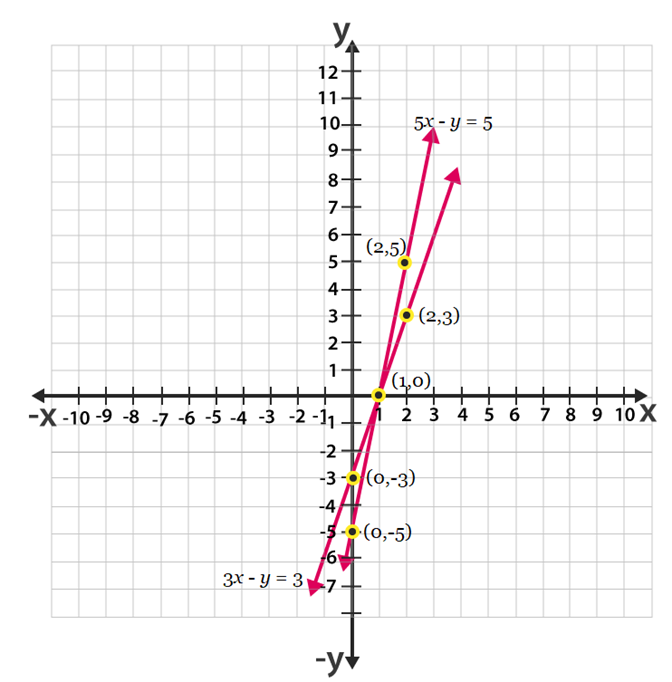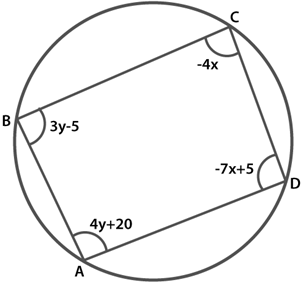### NCERT Solutions Class 10 Maths Chapter – 10 Exercise – 3.7

NCERT Solutions For Class 10 Maths Chapter – 3 Exercise – 3.7

Q1. The ages of two friends Ani and Biju differ by 3 years Ani ‘s father Dharam is twice as old as Ani and Biju is twice as old as his sister Cathy . The ages of Cathy and Dharam dffer by 30 years Find the ages of Ani and Biju.

Solution:-

X – y = 3 (I)

X – y = -3 (ii)

Ani father age = 2x years

Biju sister Cathy age = y/2 years

2x – y/2 = 30  = 4x – y = 60 (iii)

Solving (I) and (iii) we get

x = 19  , y = 16

Solving (ii) and (iii) we get x = 21   , y = 24

Q2. One says “ Give me a hundred  friend ! I shall then become twice as rich as you “ The other replies “ If you give me ten, I shall be six times as rich as you “ Tell me what is the amount of their (respective) capital ? [ From the Bijaganita of Bhaskara II]

Solution:-

X – 2y = -300 (I)

6x – y = 70 (ii)

Solving (I) and (ii) we get x = 40   , y = 170.

Q3. A train covered a certain distance at a uniform speed If the train would have been  10 km/h faster, it would have taken 2 hours less than the scheduled time. And , if the train were slower by 10km/h It would have taken 3 hours more than the scheduled time. Find the distance covered by the train.

Solution:-

Y2 + 10y – 5x = 0 (I)

3y2 – 30y – 10x = 0 (ii)

On solving equations (I) and (ii) we will obtain x = 600 km.

Q4. The students of a class are made to stand in rows. If 3 students are extra in a row there would be 1 row less. If 3 students are less in a row, there would be 2 rows more. Find the number of students in the class.

Solution:-

Total number of students = xy

(x+3)(y-1)=xy

-x + 3y = 3 (ii)

(x-3)(y+2)=xy

2x – 3y = 6 (iii)

Solving (ii) and (iii) we get

X = 9  , y = 4

5. In a ∆ABC, ∠ C = 3 ∠ B = 2 (∠A + ∠ B). Find the three angles.

Solution:

Given,

∠C = 3 ∠B = 2(∠B + ∠A)

∠B = 2 ∠A+2 ∠B

∠B=2 ∠A

∠A – ∠B= 0- – – – – – – – – – – –  (i)

We know, the sum of all the interior angles of a triangle is 180O.

Thus, ∠ A +∠B+ ∠C = 180O

∠A + ∠B +3 ∠B = 180O

∠A + 4 ∠B = 180O– – – – – – – – – – – – – – -(ii)

Multiplying 4 to  equation (i) , we get

8 ∠A – 4 ∠B = 0- – – – – – – – – – – – (iii)

Adding equations (iii) and (ii) we get

9 ∠A = 180O

∠A = 20O

Using this in equation (ii), we get

20O+ 4∠B = 180O

∠B = 40O

3∠B =∠C

∠C = 3 x 40 = 120O

Therefore, ∠A = 20O

∠B=40O

∠C = 120O

Q6. Draw the graphs of the equation5x – y = 5 and  3x – y = 3 Determine the co – ordinates of the vertices of the triangle formed by  these lines and the y axis.

Solution:-

5x – y = 5

=> y = 5x  – 5

Its solution table will be.

 x 2 1 0 y 5 0 -5

Also given , 3x – y = 3

Y = 3x – 3

 x 2 1 0 y 5 0 -5

The graphical representation of these lines will be as follows:Q7. Solve the following pair of linear equations:

• Px + qy = p – q

Qx – py = p +  q

• Ax + by = c

Bx + ay = 1 + c

• X/a – y/b = 0

Ax + by = a2 + b2

• (a – b)x + (a + b)y = a2– 2ab – b2

(a + b)(x + y) = a2 + b2

• 152x – 378y = -74

-378x + 152y = -604

Solution:-

• Px + qy = p – q (I)

qx – py = p + q (ii)

Multiplying p to equation (1) and q to equation (2) we get

P2x + pqy = p2 – pq    (iii)

q2x – pqy = pq + q2 (iv)

Adding equation (iii) and equation (iv) we get

P2x + pqy = p2  + q2

(p2 + q2 )x = p2 + q2

X = (p2 + q2) / p2 +q2 = 1

From equation (I) we get

P(1) + qy = p – q

qy = p – q – p

Y = -1

• Ax + by = c (I)

Bx + ay  = 1 + c (ii)

Multiplying a to equation (I) and b to equation (ii), we obtain

A2x + aby = ac (iii)

B2x + aby = b + bc    (iv)

Subtracting equation (iv) from equation (iii)

(a2 – b2)x = ac – bc – b

X = (ac – bc – b)/ (a2 – b2)

X = c(a – b) – / (a2 + b2)

From equation (I) we obtain

Ax + by = c

A{c(a – b)  – b)/(a2 – b2)} + by = c

By = abc – b2c + ab/a2 – b2

Y = c(a – b) + a/a2-b2

• X/a – y/b = 0 (I)

X + by = a2 + b2 (ii)

Multiplying a and b to equation (I) and (ii) respectively we get

B2x = aby = 0 (iii)

A2x + aby = a3 + ab3 (iv)

Adding equations (iii) and (iv) we get

B2x + a2x = a3 + ab2

X(b2 + a2) = a(a2 + b2)x = a

Using equation (I) we get

B(a) – ay = 0

Ab – ay = 0

Ay = ab,

Y = b

• (a – b)x + (a +b)y = a2-2ab – b2  (I)

(a + b)(x + y) = a + b2 (ii)

Subtracting equation (ii) from equation(I) we get

(a – b)x  – (a +b)x = (a2 – 2ab – b2) – (a2 + b2)

X = b + a

Substituting this value in equation (I) we get

(a +b)(a-b) + y(a +b) = a2– 2ab – b2

Y = -2ab/(a +b)

• 152x – 378y = -74 (I)

76x – 189y = -37 (ii)

Using the value of x in equation (ii) we get

-189(189y – 37/76) + 76y = -302

-(189)2y + 189 x 37 + (76)2y = -302 x 76

Y = 1

Using equation (I) we get

X = (189-37)76

X = 2

8. ABCD is a cyclic quadrilateral (see Fig. 3.7). Find the angles of the cyclic quadrilateral.Solution:

It is known that the sum of the opposite angles of a cyclic quadrilateral is 180o

Thus, we have

∠C +∠A = 180

4y + 20− 4x = 180

− 4x + 4y = 160

x − y = − 40 ……………(1)

And, ∠B + ∠D = 180

3y − 5 − 7x + 5 = 180

− 7x + 3y = 180 ………..(2)

Multiplying 3 to equation (1), we get

3x − 3y = − 120 ………(3)

Adding equation (2) to equation (3), we get

− 7x + 3x = 180 – 120

− 4x = 60

x = −15

Substituting this value in equation (i), we get

x − y = − 40

-y−15 = − 40

y = 40-15

= 25

∠A = 4y + 20 = 20+4(25) = 120°

∠B = 3y − 5 = − 5+3(25) = 70°

∠C = − 4x = − 4(− 15) = 60°

∠D = 5-7x

∠D= 5− 7(−15) = 110°

Hence, all the angles are measured.

NCERT Solutions For Class 10 Maths Chapter – 3 Exercise – 3.7   Q1. The ages of two friends Ani and…